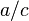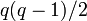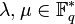# Nontrivial irreducible component of permutation representation of projective general linear group of degree two on projective line

Let$K$ be a field. Consider the projective general linear group of degree two$PGL(2,K)$. This has a natural action on the projective line over$K$, i.e., the collection of one-dimensional subspaces of the two-dimensional vector space$K^2$. We thus get a permutation representation of$PGL(2,K)$ on the projective line$\mathbb{P}^1(k)$.

The action could be described in either of these ways:

• For any element of$PGL(2,K)$, lift it to an element of$GL(2,K)$, and consider the image of any one-dimensional subspace under the element of$GL(2,K)$. Note that the image subspace does not depend on the choice of the lift, because any two lifts differ multiplicatively by a scalar matrix, which sends every subspace to itself.
• Think of$\mathbb{P}^1(K)$ as$K \cup \{ \infty\}$. For an element of$PGL(2,K)$, consider a matrix$\begin{pmatrix} a & b \\ c & d \\\end{pmatrix}$ that is a lift of this element. The permutation induced by this is the map$z \mapsto (az + b)/(cz + d)$, where the value is taken to be$\infty$ if the denominator becomes$0$, and the image of$\infty$ is taken to be$a/c$ if$c \ne 0$ and to be$\infty$ if$c = 0$.

When$K$ is a finite field of size$q$, then this gives a permutation action of a finite group$PGL(2,q)$ on a finite set of size$q + 1$. View this as a linear representation in any characteristic not dividing the order of$PGL(2,q)$. This linear representation splits as a direct sum of a trivial representation and a nontrivial irreducible representation of degree$q + 1 - 1 = q$. Our goal here is to discuss this irreducible component.

## Summary

Item Value
Degree of representation$q$
Schur index 1 in all characteristics (because the representation, being a direct summand of a permutation representation, can be realized with integer entries and hence interpreted in any characteristic).
Kernel of representation trivial subgroup. In other words, it is a faithful linear representation.
Quotient on which it descends to a faithful representation projective general linear group of degree two
Set of character values$\{ q,1,0,-1\}$
Characteristic zero: Ring generated:$\mathbb{Z}$ -- ring of integers, Ideal within ring generated: whole ring, Field generated:$\mathbb{Q}$ -- [[field of rational
Ring of realization Realized over any unital ring, by composing the representation over$\mathbb{Z}$ with the map induced by the natural homomorphism from$\mathbb{Z}$ to that ring.
Minimal ring of realization (characteristic zero)$\mathbb{Z}$ -- ring of integers
Minimal ring of realization in characteristic$p^k$ The ring of integers mod$p^k$,$\mathbb{Z}/p^k\mathbb{Z}$
Minimal field of realization Prime field in all cases.
In characteristic zero,$\mathbb{Q}$; in characteristic$p$, the field$\mathbb{F}_p$
Size of equivalence class under automorphisms 1
Size of equivalence class under Galois automorphisms 1
Size of equivalence class under action of one-dimensional representations by multiplication 2 if the characteristic of K is not 2, 1 if the characteristic of K is 2.

## Particular cases

Field size$q$ Underlying prime$p$ Group$PGL(2,q)$ Order Information on linear representation theory Description of the representation
2 2 symmetric group:S3 6 linear representation theory of symmetric group:S3 standard representation of symmetric group:S3
3 3 symmetric group:S4 24 linear representation theory of symmetric group:S4 standard representation of symmetric group:S4
4 2 alternating group:A5 60 linear representation theory of alternating group:A5 standard representation of alternating group:A5
5 5 symmetric group:S5 120 linear representation theory of symmetric group:S5 one of the five-dimensional irreducible representations

## Character

FACTS TO CHECK AGAINST (for characters of irreducible linear representations over a splitting field):
Orthogonality relations: Character orthogonality theorem | Column orthogonality theorem
Separation results (basically says rows independent, columns independent): Splitting implies characters form a basis for space of class functions|Character determines representation in characteristic zero
Numerical facts: Characters are cyclotomic integers | Size-degree-weighted characters are algebraic integers
Character value facts: Irreducible character of degree greater than one takes value zero on some conjugacy class| Conjugacy class of more than average size has character value zero for some irreducible character | Zero-or-scalar lemma

### Character values and interpretations

Nature of conjugacy class upstairs in$GL(2,q)$ Eigenvalues Characteristic polynomial Minimal polynomial Size of conjugacy class Number of such conjugacy classes Total number of elements Character value Explanation (character value = number of fixed subspaces - 1)
Diagonalizable over$\mathbb{F}_q$ with equal diagonal entries, hence a scalar$\{ a, a \}$ where$a \in \mathbb{F}_q^\ast$$(x - a)^2$ where$a \in \mathbb{F}_q^\ast$$x - a$ where$a \in \mathbb{F}_q^\ast$ 1 1 1$q$ Fixes all$q + 1$ subspaces, so character is$q + 1 - 1 = q$
Diagonalizable over$\mathbb{F}_{q^2}$, not over$\mathbb{F}_q$, eigenvalues are negatives of each other. Pair of mutually negative conjugate elements of$\mathbb{F}_{q^2}$. All such pairs identified.$x^2 - \mu$,$\mu$ a nonzero non-square Same as characteristic polynomial$q(q - 1)/2$ 1$q(q - 1)/2$ -1 No eigenvalues over$\mathbb{F}_q$, so no fixed subspaces, so character value is$0 - 1 = -1$
Diagonalizable over$\mathbb{F}_q$ with mutually negative diagonal entries.$\{ \lambda, - \lambda \}$, all such pairs identified.$x^2 - \lambda^2$, all identified Same as characteristic polynomial$q(q + 1)/2 = (q^2 + q)/2$ 1$q(q + 1)/2 = (q^2 + q)/2$ 1 Two distinct one-dimensional eigenspaces, so we get$2 - 1 = 1$.
Diagonalizable over$\mathbb{F}_{q^2}$, not over$\mathbb{F}_q$, eigenvalues are not negatives of each other. Pair of conjugate elements of$\mathbb{F}_{q^2}$. Each pair identified with anything obtained by multiplying both elements of it by an element of$\mathbb{F}_q$.$x^2 - ax + b$,$a \ne 0$, irreducible; with identification. Same as characteristic polynomial$q(q - 1)$$(q - 1)/2$$q(q -1)^2/2 = (q^3 - 2q^2 + q)/2$ -1 No eigenspaces, so we get$0 - 1 = -1$.
Not diagonal, has Jordan block of size two$a \in\mathbb{F}_q^\ast$ (multiplicity 2). Each conjugacy class has one representative of each type.$(x - a)^2$ Same as characteristic polynomial$q^2 - 1$ 1$q^2 - 1$ 0 Unique one-dimensional eigenspace, so we get$1 - 1 = 0$
Diagonalizable over$\mathbb{F}_q$ with distinct diagonal entries whose sum is not zero.$\lambda, \mu$ where$\lambda,\mu \in \mathbb{F}_q^\ast$ and$\lambda + \mu \ne 0$. The pairs$\{ \lambda, \mu \}$ and$\{ a\lambda, a\mu \}$ are identified.$x^2 - (\lambda + \mu)x + \lambda\mu$, again with identification. Same as characteristic polynomial.$q(q + 1)$$(q - 3)/2$$q(q+1)(q - 3)/2 = (q^3 - 2q^2 - 3q)/2$ 1 Two one-dimensional eigenspaces, so we get$2 - 1 = 1$.
Total NA NA NA NA$q + 2$$q^3 - q$